## Ions and Ionic Bonds

Ionic bonds are attractions between oppositely charged atoms or groups of atoms where electrons are donated and accepted.

### Learning Objectives

Predict whether a given element will more likely form a cation or an anion

### Key Takeaways

#### Key Points

• Ions form from elements when they gain or lose an electron causing the number of protons to be unequal to the number of electrons, resulting in a net charge.
• If there are more electrons than protons (from an element gaining one or more electrons), the ion is negatively charged and called an anion.
• If there are more protons than electrons (via loss of electrons), the ion is positively charged and is called a cation.
• Ionic bonds result from the interaction between a positively charged cation and a negatively charged anion.

#### Key Terms

• ion: An atom, or group of atoms, bearing an electrical charge, such as the sodium and chlorine atoms in a salt solution.
• ionic bond: A strong chemical bond caused by the electrostatic attraction between two oppositely charged ions.

### Ions and Ionic Bonds

Some atoms are more stable when they gain or lose an electron (or possibly two) and form ions. This results in a full outermost electron shell and makes them energetically more stable. Now, because the number of electrons does not equal the number of protons, each ion has a net charge. Cations are positive ions that are formed by losing electrons (as the number of protons is now greater than the number of electrons). Negative ions are formed by gaining electrons and are called anions (wherein there are more electrons than protons in a molecule ). Anions are designated by their elemental name being altered to end in “-ide”. For example, the anion of chlorine is called chloride, and the anion of sulfur is called sulfide.

This movement of electrons from one element to another is referred to as electron transfer. As illustrated, sodium (Na) only has one electron in its outer electron shell. It takes less energy for sodium to donate that one electron than it does to accept seven more electrons to fill the outer shell. When sodium loses an electron, it will have 11 protons, 11 neutrons, and only 10 electrons. This leaves it with an overall charge of +1 since there are now more protons than electrons. It is now referred to as a sodium ion. Chlorine (Cl) in its lowest energy state (called the ground state) has seven electrons in its outer shell. Again, it is more energy efficient for chlorine to gain one electron than to lose seven. Therefore, it tends to gain an electron to create an ion with 17 protons, 17 neutrons, and 18 electrons. This gives it a net charge of -1 since there are now more electrons than protons. It is now referred to as a chloride ion. In this example, sodium will donate its one electron to empty its shell, and chlorine will accept that electron to fill its shell. Both ions now satisfy the octet rule and have complete outer shells. These transactions can normally only take place simultaneously; in order for a sodium atom to lose an electron, it must be in the presence of a suitable recipient like a chlorine atom.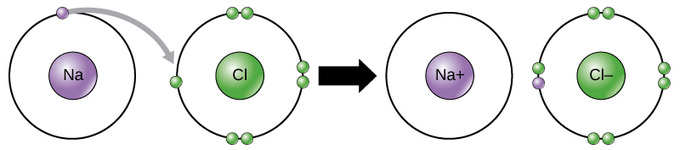Electron Transfer Between Na and Cl: In the formation of an ionic compound, metals lose electrons and nonmetals gain electrons to achieve an octet. In this example, sodium loses one electron to empty its shell and chlorine accepts that electron to fill its shell.

Ionic bonds are formed between ions with opposite charges. For instance, positively charged sodium ions and negatively charged chloride ions bond together to form sodium chloride, or table salt, a crystalline molecule with zero net charge. The attractive force holding the two atoms together is called the electromagnetic force and is responsible for the attraction between oppositely charged ions.

Certain salts are referred to in physiology as electrolytes (including sodium, potassium, and calcium). Electrolytes are ions necessary for nerve impulse conduction, muscle contractions, and water balance. Many sports drinks and dietary supplements provide these ions to replace those lost from the body via sweating during exercise.

## Covalent Bonds and Other Bonds and Interactions

Covalent bonds result from a sharing of electrons between two atoms and hold most biomolecules together.

### Learning Objectives

Compare the relative strength of different types of bonding interactions

### Key Takeaways

#### Key Points

• A polar covalent bond arises when two atoms of different electronegativity share two electrons unequally.
• A non-polar covalent bond is one in which the electrons are shared equally between two atoms.
• Hydrogen bonds and Van Der Waals are responsible for the folding of proteins, the binding of ligands to proteins, and many other processes between molecules.

#### Key Terms

• hydrogen bond: A weak bond in which a hydrogen atom in one molecule is attracted to an electronegative atom (usually nitrogen or oxygen) in the same or different molecule.
• covalent bond: A type of chemical bond where two atoms are connected to each other by the sharing of two or more electrons.
• dipole: Any object (such as a magnet, polar molecule or antenna), that is oppositely charged at two points (or poles).

### Covalent Bonds and Other Bonds and Interactions

The octet rule can be satisfied by the sharing of electrons between atoms to form covalent bonds. These bonds are stronger and much more common than are ionic bonds in the molecules of living organisms. Covalent bonds are commonly found in carbon-based organic molecules, such as DNA and proteins. Covalent bonds are also found in inorganic molecules such as H2O, CO2, and O2. One, two, or three pairs of electrons may be shared between two atoms, making single, double, and triple bonds, respectively. The more covalent bonds between two atoms, the stronger their connection. Thus, triple bonds are the strongest.

The strength of different levels of covalent bonding is one of the main reasons living organisms have a difficult time in acquiring nitrogen for use in constructing nitrogenous molecules, even though molecular nitrogen, N2, is the most abundant gas in the atmosphere. Molecular nitrogen consists of two nitrogen atoms triple bonded to each other. The resulting strong triple bond makes it difficult for living systems to break apart this nitrogen in order to use it as constituents of biomolecules, such as proteins, DNA, and RNA.

The formation of water molecules is an example of covalent bonding. The hydrogen and oxygen atoms that combine to form water molecules are bound together by covalent bonds. The electron from the hydrogen splits its time between the incomplete outer shell of the hydrogen atom and the incomplete outer shell of the oxygen atom. In return, the oxygen atom shares one of its electrons with the hydrogen atom, creating a two-electron single covalent bond. To completely fill the outer shell of oxygen, which has six electrons in its outer shell, two electrons (one from each hydrogen atom) are needed. Each hydrogen atom needs only a single electron to fill its outer shell, hence the well-known formula H2O. The electrons that are shared between the two elements fill the outer shell of each, making both elements more stable.

### Polar Covalent Bonds

There are two types of covalent bonds: polar and nonpolar. In a polar covalent bond, the electrons are unequally shared by the atoms because they are more attracted to one nucleus than the other. The relative attraction of an atom to an electron is known as its electronegativity: atoms that are more attracted to an electron are considered to be more electronegative. Because of the unequal distribution of electrons between the atoms of different elements, a slightly positive (δ+) or slightly negative (δ-) charge develops. This partial charge is known as a dipole; this is an important property of water and accounts for many of its characteristics. The dipole in water occurs because oxygen has a higher electronegativity than hydrogen, which means that the shared electrons spend more time in the vicinity of the oxygen nucleus than they do near the nucleus of the hydrogen atoms.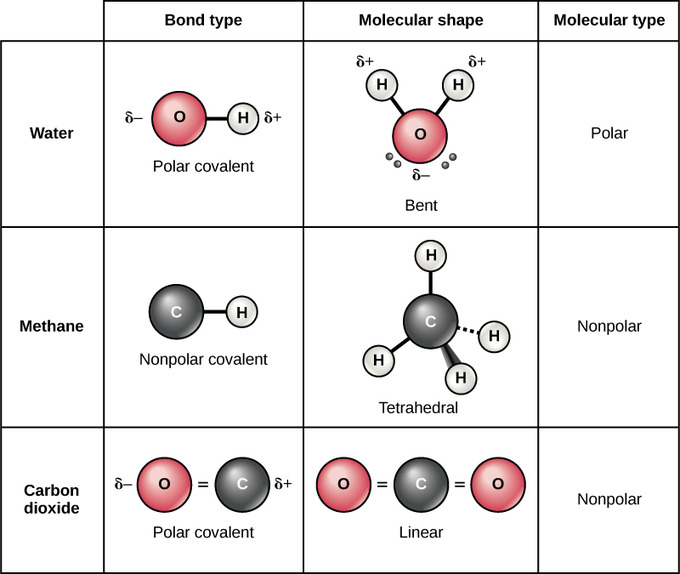Polar and Nonpolar Covalent Bonds: Whether a molecule is polar or nonpolar depends both on bond type and molecular shape. Both water and carbon dioxide have polar covalent bonds, but carbon dioxide is linear, so the partial charges on the molecule cancel each other out.

### Nonpolar Covalent Bonds

Nonpolar covalent bonds form between two atoms of the same element or between different elements that share electrons equally. For example, molecular oxygen (O2) is nonpolar because the electrons will be equally distributed between the two oxygen atoms. The four bonds of methane are also considered to be nonpolar because the electronegativies of carbon and hydrogen are nearly identical.

### Hydrogen Bonds and Van Der Waals Interactions

Not all bonds are ionic or covalent; weaker bonds can also form between molecules. Two types of weak bonds that frequently occur are hydrogen bonds and van der Waals interactions. Without these two types of bonds, life as we know it would not exist.

Hydrogen bonds provide many of the critical, life-sustaining properties of water and also stabilize the structures of proteins and DNA, the building block of cells. When polar covalent bonds containing hydrogen are formed, the hydrogen atom in that bond has a slightly positive charge (δ+) because the shared electrons are pulled more strongly toward the other element and away from the hydrogen atom. Because the hydrogen has a slightly positive charge, it’s attracted to neighboring negative charges. The weak interaction between the δ+ charge of a hydrogen atom from one molecule and the δ- charge of a more electronegative atom is called a hydrogen bond. Individual hydrogen bonds are weak and easily broken; however, they occur in very large numbers in water and in organic polymers, and the additive force can be very strong. For example, hydrogen bonds are responsible for zipping together the DNA double helix.Adenosine Triphosphate, ATP: Adenosine Triphosphate, or ATP, is the most commonly used cofactor in nature. Its biosynthesis involves the fixation of nitrogen to provide feedstocks that eventually produce the carbon-nitrogen bonds it contains.

Like hydrogen bonds, van der Waals interactions are weak interactions between molecules. Van der Waals attractions can occur between any two or more molecules and are dependent on slight fluctuations of the electron densities, which can lead to slight temporary dipoles around a molecule. For these attractions to happen, the molecules need to be very close to one another. These bonds, along with hydrogen bonds, help form the three-dimensional structures of the proteins in our cells that are required for their proper function.

Interactions between different types of molecules: In this interactive, you can explore how different types of molecules interact with each other based on their bonds.

## Hydrogen Bonding

A hydrogen bond is a strong intermolecular force created by the relative positivity of hydrogen atoms.

### Learning Objectives

Describe the properties of hydrogen bonding.

### Key Takeaways

#### Key Points

• Hydrogen bonds are strong intermolecular forces created when a hydrogen atom bonded to an electronegative atom approaches a nearby electronegative atom.
• Greater electronegativity of the hydrogen bond acceptor will lead to an increase in hydrogen-bond strength.
• The hydrogen bond is one of the strongest intermolecular attractions, but weaker than a covalent or an ionic bond.
• Hydrogen bonds are responsible for holding together DNA, proteins, and other macromolecules.

#### Key Terms

• electronegativity: The tendency of an atom or molecule to draw electrons towards itself, form dipoles, and thus form bonds.
• hydrogen bond: The attraction between a partially positively charged hydrogen atom attached to a highly electronegative atom (such as nitrogen, oxygen, or fluorine) and another nearby electronegative atom.
• intermolecular: A type of interaction between two different molecules.

### Forming a Hydrogen Bond

A hydrogen bond is the electromagnetic attraction created between a partially positively charged hydrogen atom attached to a highly electronegative atom and another nearby electronegative atom. A hydrogen bond is a type of dipole-dipole interaction; it is not a true chemical bond. These attractions can occur between molecules (intermolecularly) or within different parts of a single molecule (intramolecularly).Hydrogen bonding in water: This is a space-filling ball diagram of the interactions between separate water molecules.

### Hydrogen Bond Donor

A hydrogen atom attached to a relatively electronegative atom is a hydrogen bond donor. This electronegative atom is usually fluorine, oxygen, or nitrogen. The electronegative atom attracts the electron cloud from around the hydrogen nucleus and, by decentralizing the cloud, leaves the hydrogen atom with a positive partial charge. Because of the small size of hydrogen relative to other atoms and molecules, the resulting charge, though only partial, is stronger. In the molecule ethanol, there is one hydrogen atom bonded to an oxygen atom, which is very electronegative. This hydrogen atom is a hydrogen bond donor.

### Hydrogen Bond Acceptor

A hydrogen bond results when this strong partial positive charge attracts a lone pair of electrons on another atom, which becomes the hydrogen bond acceptor. An electronegative atom such as fluorine, oxygen, or nitrogen is a hydrogen bond acceptor, regardless of whether it is bonded to a hydrogen atom or not. Greater electronegativity of the hydrogen bond acceptor will create a stronger hydrogen bond. The diethyl ether molecule contains an oxygen atom that is not bonded to a hydrogen atom, making it a hydrogen bond acceptor.Hydrogen bond donor and hydrogen bond acceptor: Ethanol contains a hydrogen atom that is a hydrogen bond donor because it is bonded to an electronegative oxygen atom, which is very electronegative, so the hydrogen atom is slightly positive. Diethyl ether contains an oxygen atom that is a hydrogen bond acceptor because it is not bonded to a hydrogen atom and so is slightly negative.

A hydrogen attached to carbon can also participate in hydrogen bonding when the carbon atom is bound to electronegative atoms, as is the case in chloroform (CHCl3). As in a molecule where a hydrogen is attached to nitrogen, oxygen, or fluorine, the electronegative atom attracts the electron cloud from around the hydrogen nucleus and, by decentralizing the cloud, leaves the hydrogen atom with a positive partial charge.

Interactive: Hydrogen Bonding: Explore hydrogen bonds forming between polar molecules, such as water. Hydrogen bonds are shown with dotted lines. Show partial charges and run the model. Where do hydrogen bonds form? Try changing the temperature of the model. How does the pattern of hydrogen bonding explain the lattice that makes up ice crystals?

### Applications for Hydrogen Bonds

Hydrogen bonds occur in inorganic molecules, such as water, and organic molecules, such as DNA and proteins. The two complementary strands of DNA are held together by hydrogen bonds between complementary nucleotides (A&T, C&G). Hydrogen bonding in water contributes to its unique properties, including its high boiling point (100 °C) and surface tension.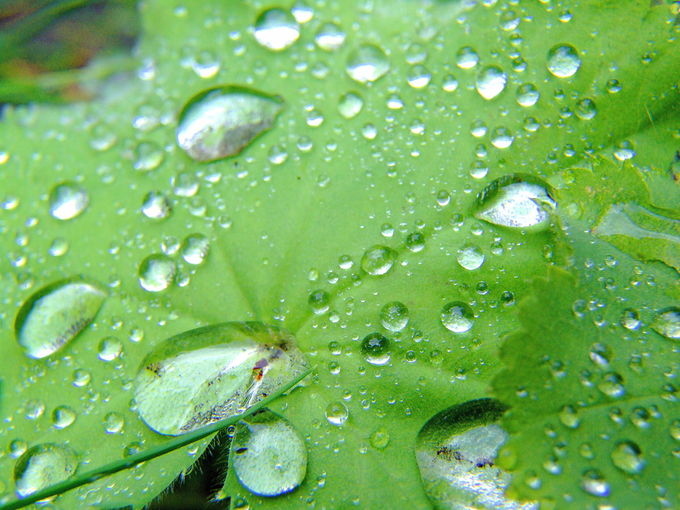Water droplets on a leaf: The hydrogen bonds formed between water molecules in water droplets are stronger than the other intermolecular forces between the water molecules and the leaf, contributing to high surface tension and distinct water droplets.

In biology, intramolecular hydrogen bonding is partly responsible for the secondary, tertiary, and quaternary structures of proteins and nucleic acids. The hydrogen bonds help the proteins and nucleic acids form and maintain specific shapes.

## Avogadro’s Number and the Mole

The mole is represented by Avogadro’s number, which is 6.022 × 1023 atoms or molecules per mol.

### Key Takeaways

#### Key Points

• The mole allows scientists to calculate the number of elementary entities (usually atoms or molecules ) in a certain mass of a given substance.
• Avogadro’s number is an absolute number: there are 6.022 × 1023 elementary entities in 1 mole. This can also be written as 6.022 × 1023 mol-1.
• The mass of one mole of a substance is equal to that substance’s molecular weight. For example, the mean molecular weight of water is 18.015 atomic mass units (amu), so one mole of water weight 18.015 grams.

#### Key Terms

• mole: The amount of substance of a system that contains as many elementary entities as there are atoms in 12 g of carbon-12.

The chemical changes observed in any reaction involve the rearrangement of billions of atoms. It is impractical to try to count or visualize all these atoms, but scientists need some way to refer to the entire quantity. They also need a way to compare these numbers and relate them to the weights of the substances, which they can measure and observe. The solution is the concept of the mole, which is very important in quantitative chemistry.

Amadeo Avogadro first proposed that the volume of a gas at a given pressure and temperature is proportional to the number of atoms or molecules, regardless of the type of gas. Although he did not determine the exact proportion, he is credited for the idea.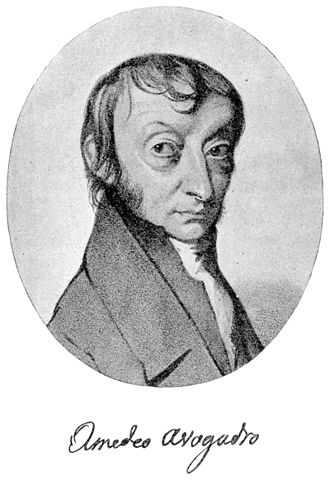Amedeo Avogadro: Amedeo Avogadro is credited with the idea that the number of entities (usually atoms or molecules) in a substance is proportional to its physical mass.

Avogadro’s number is a proportion that relates molar mass on an atomic scale to physical mass on a human scale. Avogadro’s number is defined as the number of elementary particles (molecules, atoms, compounds, etc.) per mole of a substance. It is equal to 6.022 × 1023 mol-1 and is expressed as the symbol NA.

Avogadro’s number is a similar concept to that of a dozen or a gross. A dozen molecules is 12 molecules. A gross of molecules is 144 molecules. Avogadro’s number is 6.022 × 1023 molecules. With Avogadro’s number, scientists can discuss and compare very large numbers, which is useful because substances in everyday quantities contain very large numbers of atoms and molecules.

### The Mole

The mole (abbreviated mol) is the SI measure of quantity of a “chemical entity,” such as atoms, electrons, or protons. It is defined as the amount of a substance that contains as many particles as there are atoms in 12 grams of pure carbon-12. So, 1 mol contains 6.022×1023 elementary entities of the substance.

### Chemical Computations with Avogadro’s Number and the Mole

Avogadro’s number is fundamental to understanding both the makeup of molecules and their interactions and combinations. For example, since one atom of oxygen will combine with two atoms of hydrogen to create one molecule of water (H2O), one mole of oxygen (6.022 × 1023 of O atoms) will combine with two moles of hydrogen (2 × 6.022 × 1023 of H atoms) to make one mole of H2O.

Another property of Avogadro’s number is that the mass of one mole of a substance is equal to that substance’s molecular weight. For example, the mean molecular weight of water is 18.015 atomic mass units (amu), so one mole of water weight 18.015 grams. This property simplifies many chemical computations.

If you have 1.25 grams of a molecule with molecular weight of 134.1 g/mol, how many moles of that molecule do you have?

$1.25\text{g} \times \frac{ 1 \text{ mole}}{134.1\text{g}}=0.0093 \text{ moles}.$

The Mole, Avogadro: This video introduces counting by mass, the mole, and how it relates to atomic mass units (AMU) and Avogadro’s number.

## Average Atomic Mass

The average atomic mass of an element is the sum of the masses of its isotopes, each multiplied by its natural abundance.

### Learning Objectives

Calculate the average atomic mass of an element given its isotopes and their natural abundance

### Key Takeaways

#### Key Points

• An element can have differing numbers of neutrons in its nucleus, but it always has the same number of protons. The versions of an element with different neutrons have different masses and are called isotopes.
• The average atomic mass for an element is calculated by summing the masses of the element’s isotopes, each multiplied by its natural abundance on Earth.
• When doing any mass calculations involving elements or compounds, always use average atomic mass, which can be found on the periodic table.

#### Key Terms

• mass number: The total number of protons and neutrons in an atomic nucleus.
• natural abundance: The abundance of a particular isotope naturally found on the planet.
• average atomic mass: The mass calculated by summing the masses of an element’s isotopes, each multiplied by its natural abundance on Earth.

The atomic number of an element defines the element’s identity and signifies the number of protons in the nucleus of one atom. For example, the element hydrogen (the lightest element) will always have one proton in its nucleus. The element helium will always have two protons in its nucleus.

### Isotopes

Atoms of the same element can, however, have differing numbers of neutrons in their nucleus. For example, stable helium atoms exist that contain either one or two neutrons, but both atoms have two protons. These different types of helium atoms have different masses (3 or 4 atomic mass units ), and they are called isotopes. For any given isotope, the sum of the numbers of protons and neutrons in the nucleus is called the mass number. This is because each proton and each neutron weigh one atomic mass unit (amu). By adding together the number of protons and neutrons and multiplying by 1 amu, you can calculate the mass of the atom. All elements exist as a collection of isotopes. The word ‘isotope’ comes from the Greek ‘isos’ (meaning ‘same’) and ‘topes’ (meaning ‘place’) because the elements can occupy the same place on the periodic table while being different in subatomic construction.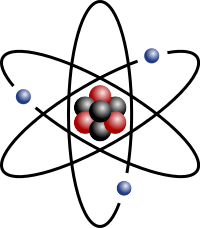Lithium Atom: Stylized lithium-7 atom: 3 protons (red), 4 neutrons (black), and 3 electrons (blue). (Lithium also has another, rarer isotope with only 2 neutrons.)

### Calculating Average Atomic Mass

The average atomic mass of an element is the sum of the masses of its isotopes, each multiplied by its natural abundance (the decimal associated with percent of atoms of that element that are of a given isotope).

Average atomic mass = f1M1 + f2M2 +… + fnMn where f is the fraction representing the natural abundance of the isotope and M is the mass number (weight) of the isotope.

The average atomic mass of an element can be found on the periodic table, typically under the elemental symbol. When data are available regarding the natural abundance of various isotopes of an element, it is simple to calculate the average atomic mass.

• For helium, there is approximately one isotope of Helium-3 for every million isotopes of Helium-4; therefore, the average atomic mass is very close to 4 amu (4.002602 amu).
• Chlorine consists of two major isotopes, one with 18 neutrons (75.77 percent of natural chlorine atoms) and one with 20 neutrons (24.23 percent of natural chlorine atoms). The atomic number of chlorine is 17 (it has 17 protons in its nucleus).

To calculate the average mass, first convert the percentages into fractions (divide them by 100). Then, calculate the mass numbers. The chlorine isotope with 18 neutrons has an abundance of 0.7577 and a mass number of 35 amu. To calculate the average atomic mass, multiply the fraction by the mass number for each isotope, then add them together.

Average atomic mass of chlorine = (0.7577 $\cdot$ 35 amu) + (0.2423 $\cdot$ 37 amu) = 35.48 amu

Another example is to calculate the atomic mass of boron (B), which has two isotopes: B-10 with 19.9% natural abundance, and B-11 with 80.1% abundance. Therefore,

Average atomic mass of boron = (0.199$\cdot$10 amu) + (0.801$\cdot$11 amu) = 10.80 amu

Whenever we do mass calculations involving elements or compounds (combinations of elements), we always use average atomic masses.